Next: Collisions in 1-dimension Up: Conservation of momentum Previous: Rocket science

## Impulses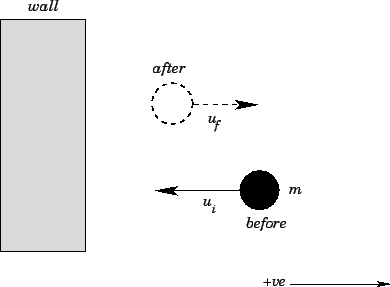Suppose that a ball of massand speed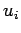strikes an immovable wall normally and rebounds with speed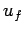. See Fig. 52. Clearly, the momentum of the ball is changed by the collision with the wall, since the direction of the ball's velocity is reversed. It follows that the wall must exert a force on the ball, since force is the rate of change of momentum. This force is generally very large, but is only exerted for the short instance in time during which the ball is in physical contact with the wall. As we have already mentioned, physicists generally refer to such a force as an impulsive force.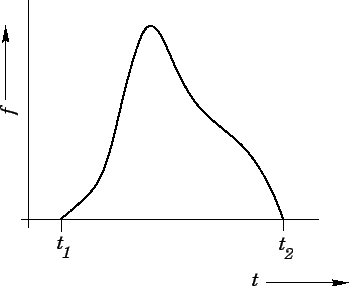Figure 53 shows the typical time history of an impulsive force,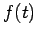. It can be seen that the force is only non-zero in the short time interval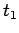to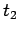. It is helpful to define a quantity known as the net impulse,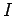, associated with: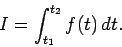(210)

In other words,is the total area under thecurve shown in Fig. 53.

Consider a object subject to the impulsive force pictured in Fig. 53. Newton's second law of motion yields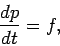(211)

where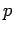is the momentum of the object. Integrating the above equation, making use of the definition (210), we obtain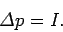(212)

Here,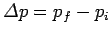, where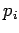is the momentum before the impulse, and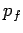is the momentum after the impulse. We conclude that the net change in momentum of an object subject to an impulsive force is equal to the total impulse associated with that force. For instance, the net change in momentum of the ball bouncing off the wall in Fig. 52 is. [Note: The initial velocity is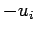, since the ball is initially moving in the negative direction.] It follows that the net impulse imparted to the ball by the wall is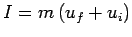. Suppose that we know the ball was only in physical contact with the wall for the short time interval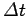. We conclude that the average force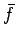exerted on the ball during this time interval was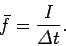(213)

The above discussion is only relevant to 1-dimensional motion. However, the generalization to 3-dimensional motion is fairly straightforward. Consider an impulsive force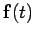, which is only non-zero in the short time intervalto. The vector impulse associated with this force is simply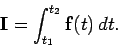(214)

The net change in momentum of an object subject tois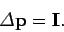(215)

Finally, if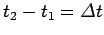, then the average force experienced by the object in the time intervaltois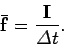(216)Next: Collisions in 1-dimension Up: Conservation of momentum Previous: Rocket science
Richard Fitzpatrick 2006-02-02